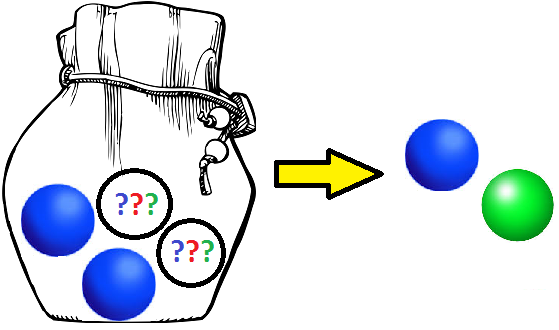# Green and Blue?

Probability Level 3

A bag holds four balls. Two are blue, and the other two each have an equiprobable chance of being red, blue or green.You reach in and pull out two balls.

If the probability of picking out a blue ball and a green ball is $\frac{a}{b}$, where $a$ and $b$ are coprime positive integers, what is $a+b$?

×

Problem Loading...

Note Loading...

Set Loading...